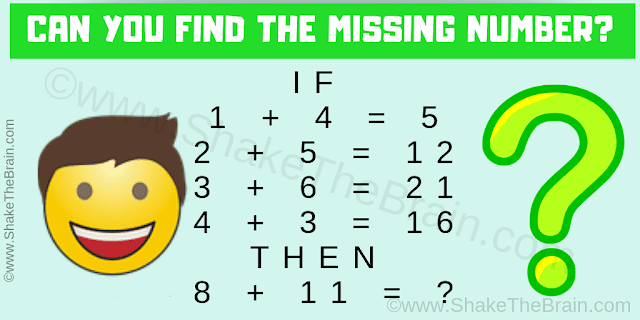## Tuesday, July 2, 2019

Here is some Logical Maths Equation. Can you find the logical pattern in these equations and then find the value of missing number which will replace the question mark?Can you solve this IQ Math Test?
Answer of this "Viral IQ Maths Test", can be viewed by clicking on the answer button.

#### 1 comment:

1.